﻿ Getting Started Tutorials - MechDesigner > Tutorial 18: Math FB > Step 18.2: Velocity: Add a Cam to the Epitrochoid Curve

# Step 18.2: Velocity: Add a Cam to the Epitrochoid Curve

### Add a Cam to the Epitrochoid Curve

We will show the difference between a 2D-Cam when:

We do enter the Velocity-Equation correctly

We do not enter the Velocity-Equation incorrectly.

A 2D-Cam is a good example, because MechDesigner must know the velocity of the Cam-Follower relative to calculate the correct Cam-Coordinates.

In this Tutorial Step, we symbolically differentiate the X and Y position equations, given in Step 18.1, to find the velocity equations.

We will enter those equations into the Math FB

MechDesigner does these calculations automatically with a Gear-Pair. We are doing this as an example of how we can use the Math FB.

#### The Parametric-Equations for the X and Y-axis Velocity Components

 Symbolically differentiate the X and Y Parametric Equations for the Epitrochoid-Curve with respect to Θ. These are the two Parametric Equations for the X and Y Velocity Components: PXvel = -(a+b)*sin(Θ) + h*((a+b)/b) * sin((a+b)/b)*Θ) PYvel = (a+b)*cos(Θ) - h*((a+b)/b) * cos((a+b)/b)*Θ) As before we must replace: a with p(0) ; b with p(1) ; h with p(2) ; Θ with p(3) In the Math FB they are: - (( p(0)+p(1))*sin(p(3))) + p(2)*(((p(0)+p(1))/p(1)))*sin(((p(0)+p(1) ) / p(1)*p(3)))  ((p(0)+p(1))*cos(p(3))) - p(2)*(((p(0)+p(1))/p(1)))*cos(((p(0)+p(1))/p(1)*p(3)))

#### Enter the Parametric Equations for the Velocity Components in the Math FB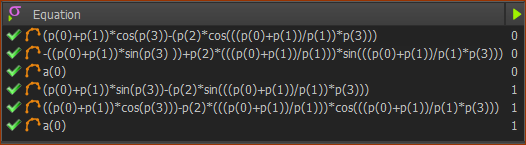Position and Velocity Equations These are the 6 equations for the two output-connectors As entered the Math FB: •Equations 1 & 2: Position and Velocity of the X -axis •Equations 4 &5: Position and Velocity for the Y-axis •Equations 3 & 6: Default - acceleration for X-axis & Y-axis respectively - not yet entered.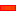Top-Tip - Cut and Paste the equations directly into the Math FB.

The two Sliders move with the parametric equations to generate the the Epitrochoid Curve

But, can we add a 2D-Cam and get the Cam-Coordinates?

We will find that if the Velocity equations are not correct, the Cam will not be correct.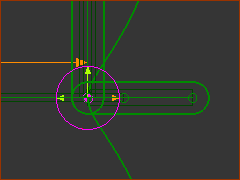Add a Cam-Follower to the Y-Slider of the Piggyback Sliders

 1.Mechanism-Editor : Edit the Y-Slider Part 2.Part-Editor : Geometry toolbar > Add Circle - Drag in the graphic-area 3.Part-Editor: Geometry toolbar > Add Dimension - Click the Circle to define its radius. 4.Part-Editor : Constraints toolbar > Coincident - Click the center-Point of the Circle and the Y-axis ; again: its center-Point and X-axis 5.Part-Editor : Exit the Part-Editor 6.Mechanism-Editor : Solids menu > Add Profile : Click the Circle, Click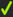in the Command-Manager.

 1.Mechanism-Editor: Click Machine element > Add 2D-Cam The Command-Manager has two selection-boxes: 2.Mechanism-Editor: Click the Base-Part as the Cam-Part 3.Mechanism-Editor: Click the Profile - the Cam-Follower 4.Mechanism-Editor: Clickin the Command-Manager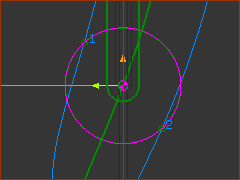Look closely at the Cam and the Cam-Follower.

 1.Run menu > Cycle or (ALT+C) , and again,  to start then stop the machine-cycle or 1.Drag the Master Machine Angle to a different Machine Angle

You can see the contact between the 2D-Cam and the Cam-Follower.

The 2D-Cam is not correct.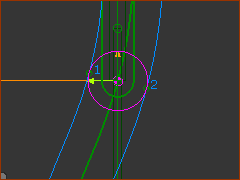Check and correct the Velocity Equations in the Math FB.

Now, the 2D-Cam is correct.

Now you can get the Cam-Coordinates in the usual way.